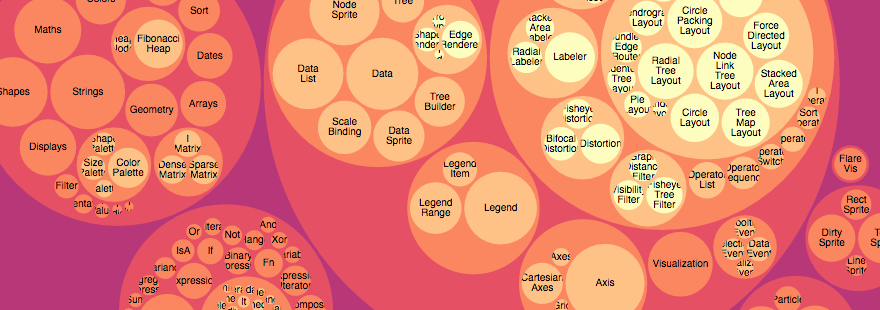# Pack ​Examples · Enclosure diagrams use containment (nesting) to represent a hierarchy. The size of the leaf circles encodes a quantitative dimension of the data. The enclosing circles show the approximate cumulative size of each subtree, but due to wasted space there is some distortion; only the leaf nodes can be compared accurately. Although circle packing does not use space as efficiently as a treemap, the “wasted” space more prominently reveals the hierarchical structure.

## pack() ​

Source · Creates a new pack layout with the default settings.

### pack(root) ​

Source · Lays out the specified root hierarchy, assigning the following properties on root and its descendants:

• node.x - the x-coordinate of the circle’s center
• node.y - the y coordinate of the circle’s center
• node.r - the radius of the circle

You must call root.sum before passing the hierarchy to the pack layout. You probably also want to call root.sort to order the hierarchy before computing the layout.

Source · If radius is specified, sets the pack layout’s radius accessor to the specified function and returns this pack layout. If radius is not specified, returns the current radius accessor, which defaults to null. If the radius accessor is null, the radius of each leaf circle is derived from the leaf node.value (computed by node.sum); the radii are then scaled proportionally to fit the layout size. If the radius accessor is not null, the radius of each leaf circle is specified exactly by the function.

## pack.size(size) ​

Source · If size is specified, sets this pack layout’s size to the specified two-element array of numbers [width, height] and returns this pack layout. If size is not specified, returns the current size, which defaults to [1, 1].

## packSiblings(circles) ​

Source · Packs the specified array of circles, each of which must have a circle.r property specifying the circle’s radius. Assigns the following properties to each circle:

• circle.x - the x-coordinate of the circle’s center
• circle.y - the y coordinate of the circle’s center

The circles are positioned according to the front-chain packing algorithm by Wang et al.

## packEnclose(circles) ​

Examples · Source · Computes the smallest circle that encloses the specified array of circles, each of which must have a circle.r property specifying the circle’s radius, and circle.x and circle.y properties specifying the circle’s center. The enclosing circle is computed using the Matoušek-Sharir-Welzl algorithm. (See also Apollonius’ Problem.)

Resources
Observable